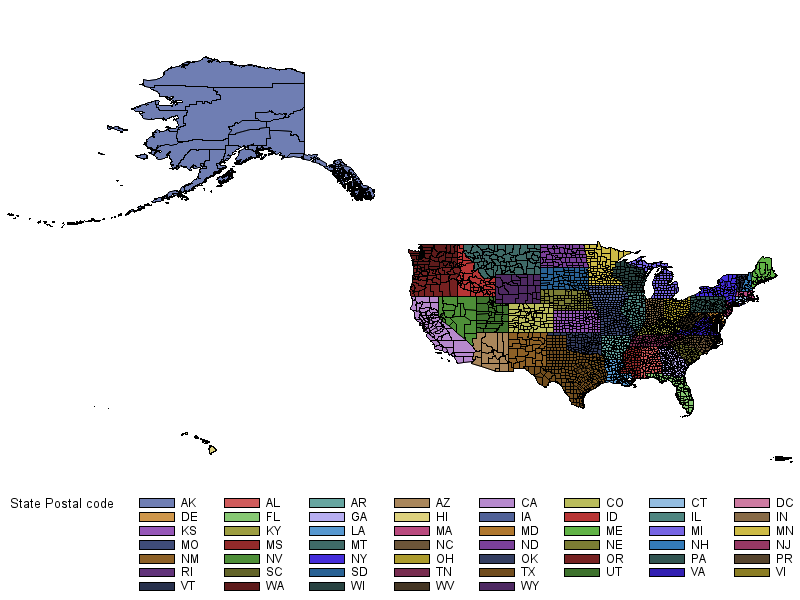## US States Heat Map including Territories

I need to create a heat map for the US and its territories. I am using this code, which is giving me what I need for the "main land", but not the territories. I am using my own data for the values that create the heat map's color for each state.

Any help?

``````

proc sort data=mapsgfk.us out=USBorders2 equals;

by statecode;

run;

proc sort data=sashelp.us_data(keep=density_2010 statecode) out=USDensity;

by statecode;

run;

data USDensity2;

merge USBorders2 USDensity;

by statecode;

if statecode ne '' then density_2010 = 100 ;

if statecode = 'IL' then density_2010 = - 20;

if statecode = 'IN' then density_2010 = 88;

if statecode = 'IA' then density_2010 = 68;

if statecode = 'KS' then density_2010 = - 20;

if statecode = 'KY' then density_2010 = 87;

if statecode = 'MI' then density_2010 = 41;

if statecode = 'MN' then density_2010 = - 20;

if statecode = 'MO' then density_2010 = - 20;

if statecode = 'NE' then density_2010 = - 20;

if statecode = 'OH' then density_2010 = 46;

if statecode = 'WI' then density_2010 = - 20;

if statecode = 'CT' then density_2010 = 90;

if statecode = 'DE' then density_2010 = 68;

if statecode = 'DC' then density_2010 = - 20;

if statecode = 'ME' then density_2010 = - 20;

if statecode = 'MD' then density_2010 = - 20;

if statecode = 'MA' then density_2010 = 59;

if statecode = 'NH' then density_2010 = - 20;

if statecode = 'NJ' then density_2010 = 53;

if statecode = 'NY' then density_2010 = 89;

if statecode = 'PA' then density_2010 = 62;

if statecode = 'RI' then density_2010 = - 20;

if statecode = 'VT' then density_2010 = - 20;

if statecode = 'AL' then density_2010 = 68;

if statecode = 'FL' then density_2010 = 49;

if statecode = 'GA' then density_2010 = - 20;

if statecode = 'NC' then density_2010 = - 20;

if statecode = 'SC' then density_2010 = - 20;

if statecode = 'TN' then density_2010 = 87;
if statecode = 'VA' then density_2010 = - 20;

if statecode = 'WV' then density_2010 = - 20;

if statecode = 'AR' then density_2010 = 52;

if statecode = 'CO' then density_2010 = 48;

if statecode = 'LA' then density_2010 = 27;

if statecode = 'MS' then density_2010 = 48;

if statecode = 'NM' then density_2010 = 77;

if statecode = 'OK' then density_2010 = 59;

if statecode = 'TX' then density_2010 = 38;

if statecode = 'ND' then density_2010 = - 20;

if statecode = 'AK' then density_2010 = 72;

if statecode = 'AS' then density_2010 = - 20;

if statecode = 'AZ' then density_2010 = 38;

if statecode = 'CA' then density_2010 = - 20;

if statecode = 'HI' then density_2010 = - 20;

if statecode = 'ID' then density_2010 = 49;

if statecode = 'MT' then density_2010 = 30;

if statecode = 'NV' then density_2010 = 43;

if statecode = 'OR' then density_2010 = 41;

if statecode = 'SD' then density_2010 = - 20;

if statecode = 'UT' then density_2010 = 48;

if statecode = 'WA' then density_2010 = 50;

if statecode = 'WY' then density_2010 = 61;

if statecode = 'VI' then density_2010 = 49;

if statecode = 'PR' then density_2010 = 83;

run;

%annomac /* Make the SAS/GRAPH annotate macros available */

%centroid(mapsgfk.us,centers2,id) /* Polygon center is used to place each label */

data map2(drop=statecode segment);

set USDensity2 centers2(rename=(x=xCen y=yCen) in=a);

if a then Label=fipstate(input(substr(id,4,2), 2.)); /* State postal code */

id = catx('-', id, segment); /* Combine ID and Segment to make unique ID */

if label = 'ID' then ycen + -.025; /* Adjust a few label coordinates */

if label = 'MI' then ycen + -.025;

if label = 'HI' then ycen + -.01;

if label = 'NH' then ycen + -.012;

if label = 'VT' then ycen + .01;

if label = 'MD' then do; ycen + -.02; xcen + .037; end;

if label = 'AK' then ycen + .01;

if label = 'DE' then do; ycen + -.005; xcen + .025; end;

if label = 'CT' then do; ycen + -.009; xcen + .032; end;

if label = 'NJ' then do; xcen + .015; end;

if label = 'RI' then do; xcen + .015; end;

if label = 'DC' then delete;

run;

options orientation=landscape;

ods graphics on / height=7.6in width=12.8in;

ods pdf file='nv_highlow.pdf' notoc dpi=300;

proc sgplot data=map2 noautolegend;

title height=5 'Heat Map, 2019';

footnote height=3 "Notes, Notes, Notes" c=gray;

footnote height=3 "State Name" c=gray;

polygon x=x y=y id=id / outline lineattrs=(color=blue)

fill colormodel=(white red white green) name='map2';

scatter x=xcen y=ycen / markerchar=label markercharattrs=(size=10);

label density_2010 = ' High Risk     Low Risk';

xaxis offsetmin=0.01 offsetmax=0.01 display=none;

yaxis offsetmin=0.01 offsetmax=0.01 display=none;

run;

ods graphics on / reset=all;``````
3 REPLIES 3

## Re: US States Heat Map including Territories

Which other 'territories' are you wanting, and where would you like for them to show up in the map?

You'll likely have to subset them from another map, and then re-scale them, and apply x/y offsets, to get them to show up 'around' the main US 48 states (like Hawaii and Alaska often are).

## Re: US States Heat Map including Territories

Just PR and VI for now.

## Re: US States Heat Map including Territories

mapsgfk.us_all contains Puerto Rico and Virgin Islands ...

proc gmap data=mapsgfk.us_all map=mapsgfk.us_all;
id id;
choro statecode;
run;Discussion stats
• 3 replies
• 758 views
• 1 like
• 2 in conversation